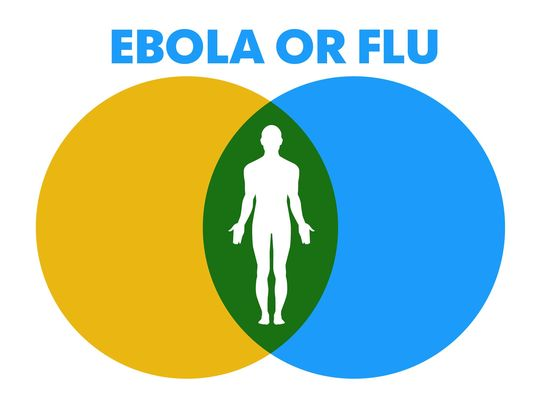# By the Numbers, Do You Have Ebola or the Flu?

The nation is receiving daily news updates on Ebola in the United States, and the debate in the public arena is heated. The mortality rate for Ebola is reported to be as high as 70 percent and there remains some reported uncertainty about the modes of transmission. Combined with the speed and ease of international travel, people may become concerned if they develop symptoms that have been associated with Ebola.

Certainly, anyone developing a fever, aches, or gastrointestinal problems should see a doctor and get diagnosed, but the greatest danger is not Ebola, it is other diseases with similar symptoms.

It is crucial to remember the following (as examples):• The probability that you have a fever if you have Ebola is very high – greater than 0.9
• The probability that you have a fever if you have the flu (for example) is also very high – greater than 80 percent
• Neither of those are informative statements. The useful question is, “If I have a fever, what is the probability that I have Ebola or the flu or some other disease?”
• In order to answer that question, it is critical to understand the prevalence of fevers in the general population and the prevalence of the various diseases (Ebola and the flu) in the general population.
• That information allows a doctor to diagnose the most likely disease (if the doctor is fluent in math, she can actually compute the probabilities).
• The driving factor is the prevalence of the disease – in the case of Ebola, the prevalence is very low (given that the doctor understands the patient’s history with regard to exposure to known Ebola patients) while the prevalence of the flu and other diseases with some of the same symptoms is much higher.

Very quickly (and using very approximate probabilities), if someone has a fever, then here is what the probabilities look like in the United States for someone who has not been to West Africa:

Influenza:

Probability of having the flu if a fever is present = (probability of a fever given that a person has the flu) x (likelihood of the flu in the general population)/(probability that a person has a fever in the general population). Using algebraic notation, Pflu= P(fever given flu)xP(flu)/P(fever). Substituting numbers, we get Pflu= (0.8 x 0.1)/0.1 = 0.8 or an 80% probability that the person has the flu.

Ebola:

Probability of having Ebola if a fever is present = (probability of a fever given that a person has Ebola) x (likelihood of Ebola in the general population)/(probability that a person has a fever in the general population). Using algebraic notation, PE=P(fever given Ebola)xP(Ebola)/P(fever). Again, substituting numbers, we get PE=(0.9 x 0.00000001)/0.1 = .0000009 or .000009%.

So, if you have a fever, the (very rough) odds that you have the flu rather than Ebola are about 90,000,000 to one in your favor.

That said, remember that thousands of Americans die each year from the flu. Remember also that the flu vaccine is only about 70 percent effective, so if you develop symptoms, you do need to see your doctor – even though you don’t have Ebola.

*photo credit USA Today